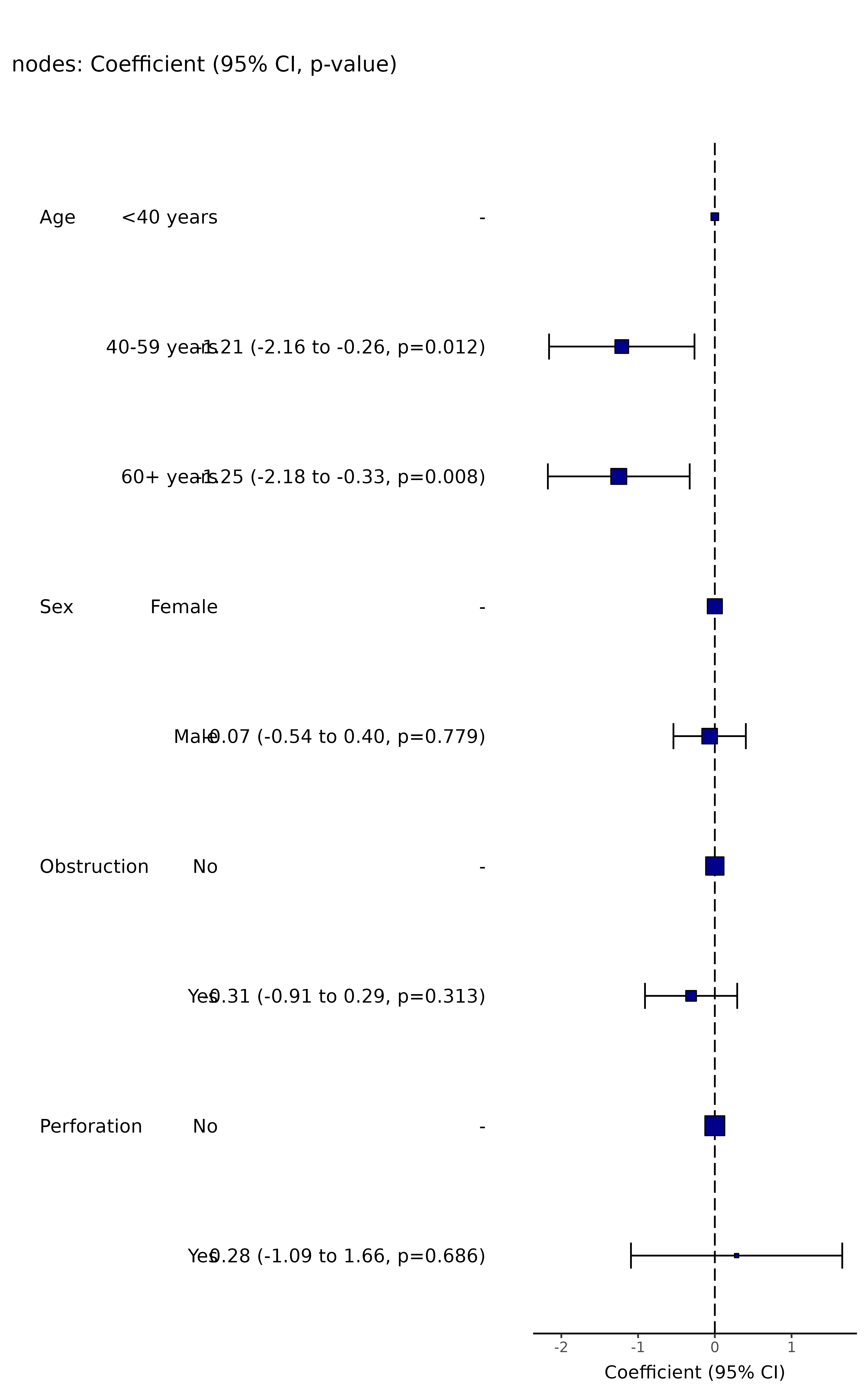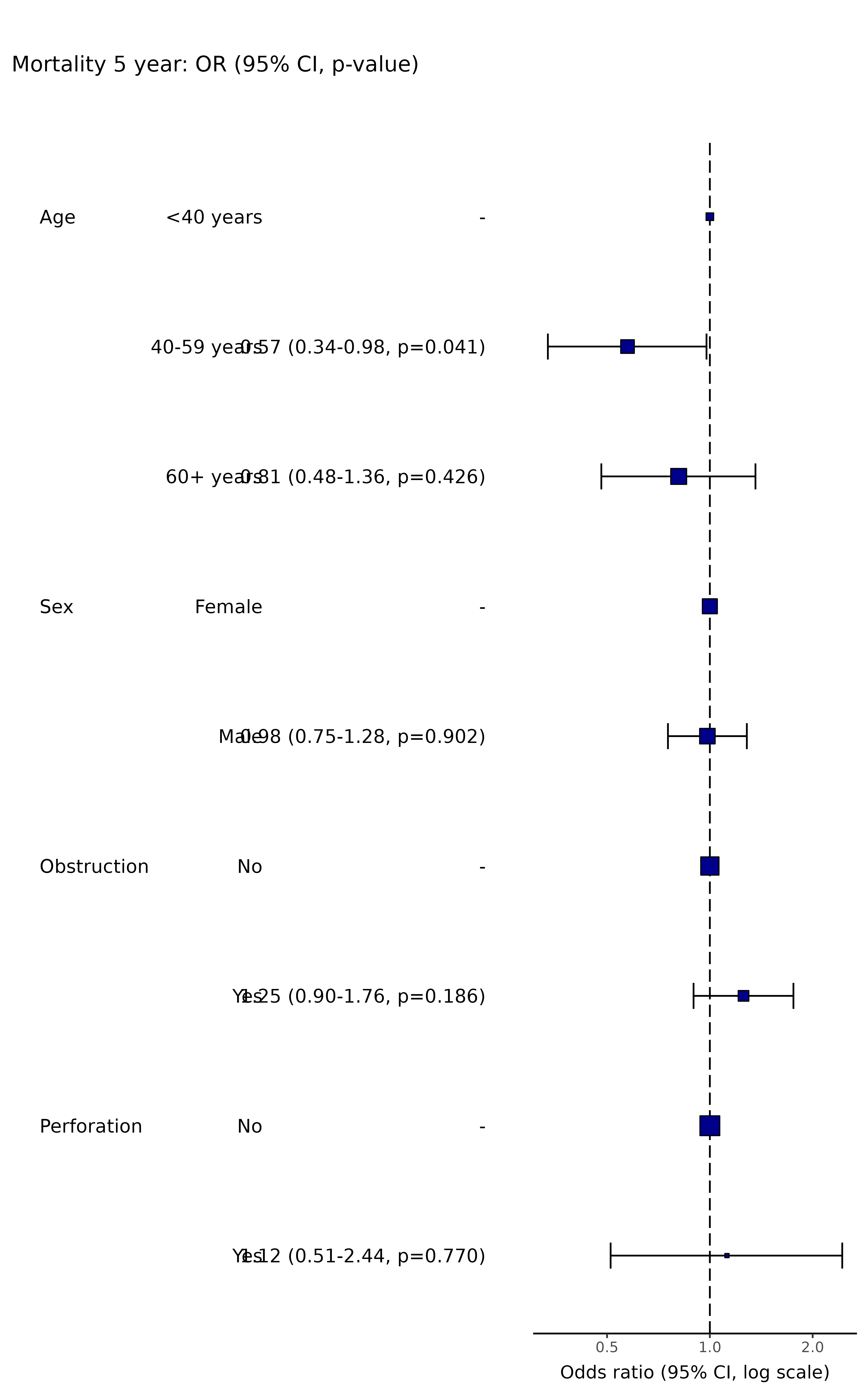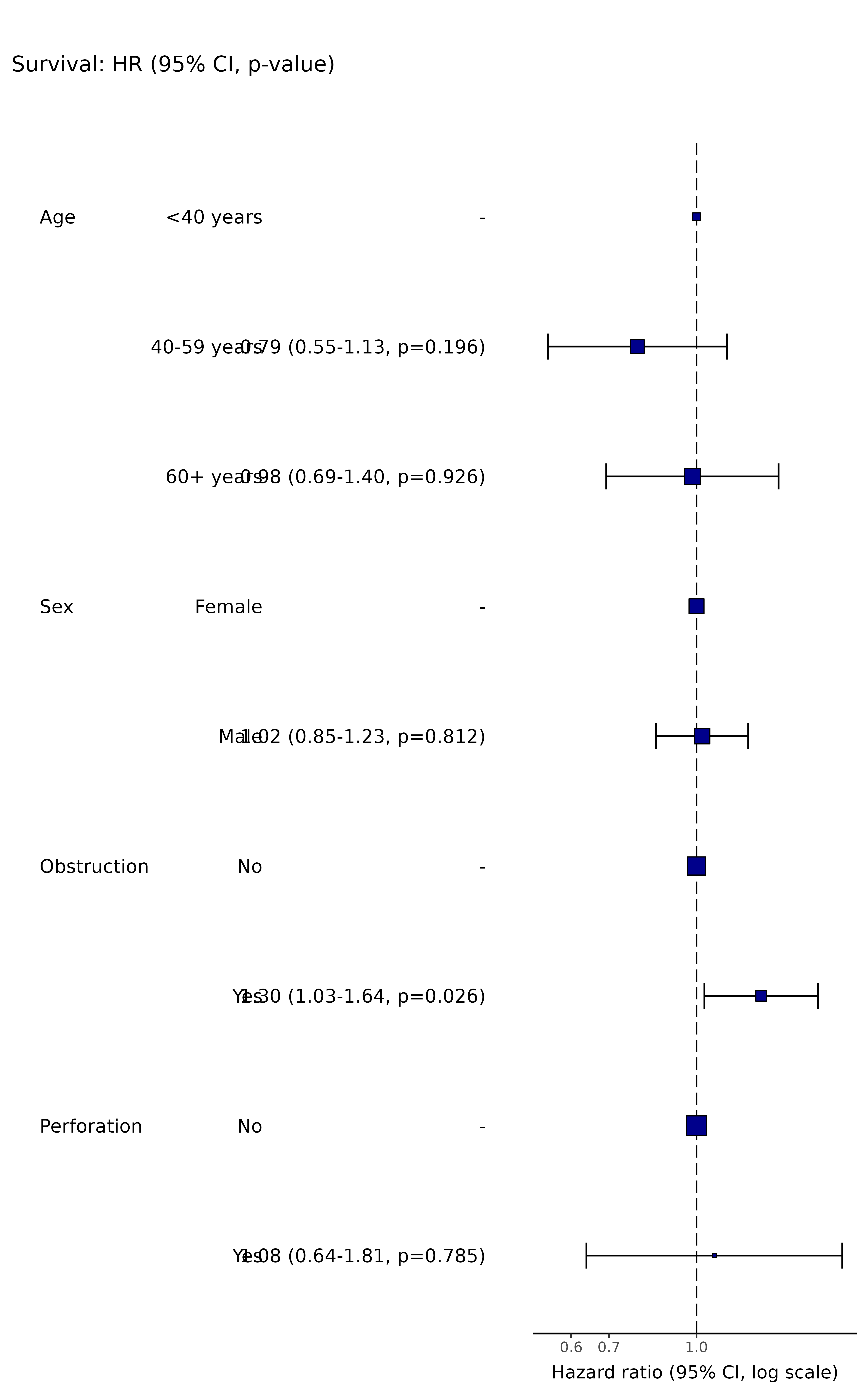Wraps or_plot, hr_plot, and coefficient_plot and sends to the appropriate method depending on the dependent variable type.

ff_plot(.data, dependent, explanatory, ...)

finalfit_plot(.data, dependent, explanatory, ...)

## Arguments

.data

Data frame.

dependent

Character vector of length 1.

explanatory

Character vector of any length: name(s) of explanatory variables.

...

Pass arguments or_plot, hr_plot, or coefficient_plot

## Value

A table and a plot using ggplot2

Other finalfit plot functions: coefficient_plot(), hr_plot(), or_plot(), surv_plot()

## Examples

# Coefficient plot
explanatory = c("age.factor", "sex.factor", "obstruct.factor", "perfor.factor")
dependent = "nodes"
colon_s %>%
ff_plot(dependent, explanatory)
#> Note: dependent includes missing data. These are dropped.
#> Warning: Removed 4 rows containing missing values (geom_errorbarh).# Odds ratio plot
dependent = "mort_5yr"
colon_s %>%
ff_plot(dependent, explanatory)
#> Note: dependent includes missing data. These are dropped.
#> Waiting for profiling to be done...
#> Waiting for profiling to be done...
#> Waiting for profiling to be done...
#> Warning: Removed 4 rows containing missing values (geom_errorbarh).# Hazard ratio plot
dependent = "Surv(time, status)"
colon_s %>%
ff_plot(dependent, explanatory, dependent_label = "Survival")
#> Dependent variable is a survival object
#> Warning: Removed 4 rows containing missing values (geom_errorbarh).